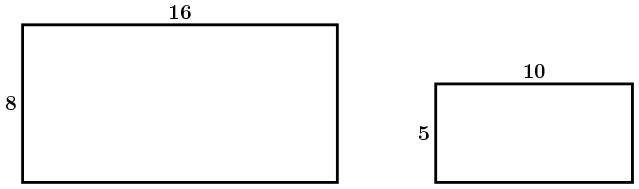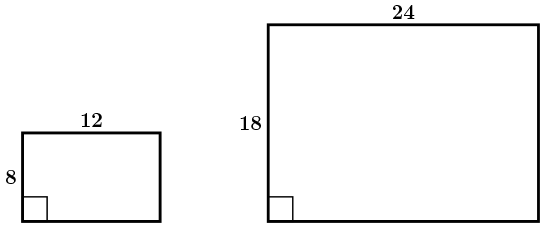# RATL 1 | Lesson 3 | Try This! (Similar Rectangles)

## Similar Rectangles

Use a ruler and cut paper rectangles with the following dimensions (all dimensions are in inches):

A) 3 by 5

B) 4 by 6

C) 6 by 8

D) 5 by 7

E) 8.5 by 11

F) 5.5 by 8.5

G) 4.25 by 5.5

1. Sort the rectangles by shape.

2. Make a list of pairs of rectangles that appear to be scaled versions of each other (or have sides that are proportional).

Two rectangles are similar if they are the exact same shape but are dissimilar in size. Also, to be similar, the rectangles must have side lengths that are proportional.

Example 1: The following two rectangles are similar.The sides lengths can be compared multiplicatively:

• The longer dimension of each rectangle is twice the measure of the shorter dimension.
• When corresponding side lengths are compared, the larger rectangle’s dimensions are 1.6 times as long as the smaller rectangle’s.

Example 2: The following two rectangles are not similar.The ratios of the sides of the rectangles are not the same meaning that the sides are not proportional.

• The ratio of the sides of the first rectangle is 2/3.
• The ratio of the sides of the second rectangle is 3/4.

3. Use ratios of the sides to determine which pairs of rectangles are similar.  How did your list compare?

A) 3 by 5

B) 4 by 6

C) 6 by 8

D) 5 by 7

E) 8.5 by 11

F) 5.5 by 8.5

G) 4.25 by 5.5

*Note: Increasing one dimension, while keeping the other the same, is not scaling, and therefore, not a multiplicative comparison. And, increasing each dimension by the same length is not scaling.

Check solutions here.